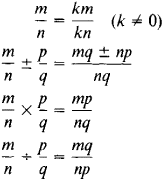# rational number

(redirected from Rational field)
Also found in: Dictionary, Thesaurus, Financial.

## rational number:

see numbernumber,
entity describing the magnitude or position of a mathematical object or extensions of these concepts. The Natural Numbers

Cardinal numbers describe the size of a collection of objects; two such collections have the same (cardinal) number of objects if their
.

## Rational Number

a number that can be expressed in the form of a fraction m/n, where m and η are integers and n ≠ 0. Since an integer m can be expressed as m/1, all integers are rational numbers. The operations of addition, subtraction, multiplication, and division (by nonzero divisors) can always be performed in the domain of rational numbers; thus, the rational numbers form a field. The basic rules of the operations over the rational numbers are given by the formulasRational numbers can also be represented in the form of finite decimals or infinite periodic decimals. Every irrational number can be included between two rational numbers, the difference between which can be made arbitrarily small.

## rational number

[′rash·ən·əl ′nəm·bər]
(mathematics)
A number which is the quotient of two integers.

## rational number

any real number of the form a/b, where a and b are integers and b is not zero, as 7 or 7/3
Site: Follow: Share:
Open / Close